## Main

Quantum confinement in graphene has previously been accomplished through lithographically patterned structures14,15,16,17, graphene edges18, and chemically synthesized graphene islands19,20,21,22. These systems, however, are either too contaminated for direct wavefunction visualization or use metallic substrates that prevent electrostatic gating. Electron confinement in graphene has also been induced through high magnetic fields23 and supercritical impurities24, but these methods are unwieldy for many technological applications. An alternative approach for confining electrons in graphene relies on using electrostatic potentials. However, this is notoriously difficult because Klein tunnelling renders electric potentials transparent to massless Dirac fermions at non-oblique incidence1,2,3,4,5. Nevertheless, it has been theoretically predicted that a circular graphene p–n junction can localize Dirac electrons and form quasi-bound quantum dot states6,7,8,9,10,11. A recent tunnelling spectroscopy experiment12 revealed signatures of electron confinement induced by the electrostatic potential created by a charged scanning tunnelling microscope (STM) tip. However, since the confining potential moves with the STM tip, this method allows neither spatial imaging of the resulting confined modes nor patterning control of the confinement potential.

Here we employ a new patterning technique that allows the creation of stationary circular p–n junctions in a graphene layer on top of hexagonal boron nitride (hBN). Figure 1a illustrates how stationary circular graphene p–n junctions are created. We start with a graphene/hBN heterostructure resting on a SiO2/Si substrate. The doped Si substrate acts as a global backgate while the hBN layer provides a tunable local embedded gate after being treated by a voltage pulse from an STM tip13. To create this embedded gate the STM tip is first retracted approximately 2 nm above the graphene surface and a voltage pulse of Vs = 5 V is then applied to the STM tip while simultaneously holding the backgate voltage to Vg = 40 V. The voltage pulse ionizes defects in the hBN region directly underneath the tip25 and the released charge migrates through the hBN to the graphene13. This leads to a local space-charge build-up in the hBN that effectively screens the backgate and functions as a negatively charged local embedded gate13 (using the opposite polarity gate voltage during this process leads to an opposite polarity space charge). Adjusting Vg afterwards allows us to tune the overall doping level so that the graphene is n-doped globally, but p-doped inside a circle centred below the location where the tip pulse occurred (it is also possible to control the charge carrier density profile as well as create opposite polarity p–n junctions by changing the Vg applied during the tip pulse). As shown schematically in Fig. 1b, the STM tip can then be moved to different locations to probe the electronic structure of the resulting stationary circular p–n junction.

To confirm that this procedure results in a circular p–n junction, we measured STM differential conductance (dI/dVs) as a function of sample bias (Vs) on a grid of points covering the graphene area near a tip pulse. The Dirac point energy, ED, was identified at every pixel, allowing us to map the charge carrier density, n, through the relation n(x, y) = −(sgn(ED)ED2)/(π(vF)2), where vF = 1.1 × 106 m s−1 is the graphene Fermi velocity and is the reduced Planck constant. Figure 1c shows the resulting n(x, y) for a tip pulse centred in the top right corner (the carrier density n can be adjusted by changing Vg). The interior blue region exhibits positive charge density (p-type) whereas the red region outside has negative charge density (n-type).

To spatially map the local electronic properties of such circular p–n junctions, we examined a rectangular sector near a p–n junction, as indicated in Fig. 2a. Figure 2b shows a topographic image of the clean graphene surface in this region. A 2.8 nm moiré pattern (corresponding to a 5° rotation angle between graphene and hBN) is visible26,27 and the region is seen to be free of adsorbates. A dI/dVs map of the same region (Fig. 2c) reflects changes in the local density of states (LDOS) caused by the spatially varying charge density distribution. Since the p–n junction centre is stationary, we are able to move the STM tip to different locations inside and outside the p–n junction to spatially resolve the resulting electronic states. Figure 2d–g shows d2I/dVs2(Vg, Vs) plots at four different locations, as denoted in Fig. 2c. We plot the derivative of dI/dVs with respect to Vs to accentuate the most salient features, which are quasi-periodic resonances that disperse to lower energies with increasing Vg (see Supplementary Section 2 for dI/dVs sweeps before differentiation). The energies of the observed resonances are seen to evolve as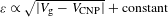, where VCNP is the local charge neutrality point, as expected for graphene’s relativistic band structure. We see that the energy spacing between observed resonances (Δɛ) decreases as we move away from the p–n junction centre until the resonances disappear outside. For example, Δɛ is 29 ± 2 mV at the centre, 16 ± 2 mV at 50 nm from the centre, and 13 ± 2 mV at 100 nm from the centre (for Vg = 32 V). A similar trend is also observed for p–n junctions that are n-doped in the centre and p-doped outside (Supplementary Section 3).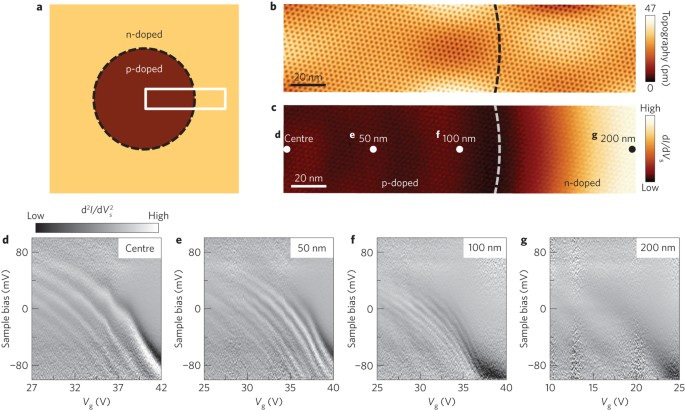Figure 2: Gate-tunable electronic structure of a circular graphene p–n junction.

We have imaged these electronic states both inside and outside of circular p–n junctions. The dI/dVs maps in Fig. 3a, b show eigenstate distributions mapped at two different energies within the same section of a circular p–n junction (similar to the boxed region of Fig. 2a, but with opposite heterojunction polarity). Circular quantum interference patterns resulting from confined Dirac fermions are clearly observed within the junction boundary, as well as scattering states exterior to the boundary. The junction boundary is demarcated by a dark band (low dI/dVs) in the middle of each dI/dVs map (and further marked by a dashed line). Comparing the overall spatial locations of the nodes and anti-nodes, the two eigenstate distributions in Fig. 3a, b are clearly different (for example, one has a node at the origin, whereas the other exhibits a central anti-node). Figure 4a shows a more complete mapping of the energy-dependent eigenstates (within a p–n junction of the same polarity as Fig. 2a) along a line extending from the centre (left edge) to a point outside of the p–n junction (right edge) at a gate voltage of Vg = 32 V. The data are plotted as d2I/dVs2(r, Vs) (where r is the radial distance from the centre) to accentuate the striking oscillatory features (see Supplementary Section 2 for dI/dVs(r, Vs) before differentiation). The energy level structure and interior nodal patterns are clearly evident.Figure 3: dI/dVs images of quantum interference throughout a circular graphene p–n junction.Figure 4: Spatially resolving energy levels inside a circular graphene p–n junction.

Our observations can be explained by considering the behaviour of massless Dirac fermions in response to a circular electrostatic potential. Due to Klein tunnelling, a graphene p–n junction perfectly transmits quasiparticles at normal incidence to the boundary, but reflects them at larger angles of incidence1,4,5. In a potential well with circular symmetry, electrons with high angular momenta are obliquely incident on the barrier and are internally reflected, thus leading to particle confinement and the formation of quasi-bound quantum dot states7,8,9,10,11,12. As angular momentum is increased, electrons are repelled from the centre of the potential by the centrifugal barrier, leading to an increase in the number of dI/dVs resonances that should be observable in spectroscopy measured away from the centre28. This is consistent with our observation that the apparent energy spacing between resonances (Δɛ) at the centre (Fig. 2d) is approximately double the apparent energy spacing at a point 100 nm away from the centre (Fig. 2f). Scattered quasiparticles (with nonzero angular momenta) external to the potential boundary contribute to Friedel oscillations that radiate outwards, as seen in Fig. 3. A circular graphene p–n junction with an n-doped interior thus acts as a quantum dot for electron-like carriers and a quantum antidot for hole-like carriers (as in Fig. 3), whereas the reverse is true for p–n junctions of opposite polarity (as in Figs 2 and 4).

This qualitative picture can be confirmed by comparing the experimental results to a model based on the two-dimensional massless Dirac Hamiltonian,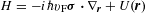, where U(r) is a scalar potential and σ = (σx, σy) are the pseudospin Pauli matrices. Since we are interested in the low-energy eigenstates of the confinement potential, we use a parabolic model U(r) = −κr2 (that is, the lowest order approximation). The curvature of the potential, κ = 6 × 10−3 meV nm−2, was extracted from measurements of the spatially dependent Dirac point energy (Supplementary Section 1). We solved the Dirac equation to obtain the eigenstates for Dirac fermions in this confinement potential (see Methods).

Figure 4b shows the results of our calculations in a plot of LDOS/∂ɛ, the energy derivative of the LDOS, which corresponds to the experimental quantity d2I/dVs2. The resulting eigenstate distribution (Fig. 4b) closely resembles the experimental eigenstate distribution (Fig. 4a). Both have a characteristic parabolic envelope due to the confinement potential, as well as a complex set of interior nodal patterns. The characteristic energy spacing seen experimentally is in good agreement with the characteristic energy scale ɛ = (2vF2κ)1/3 ≈ 15 meV that arises from the theoretical model.

Further insight into the nature of the observed resonances can be gained by directly comparing constant-energy experimental dI/dVs line-cuts (Fig. 4c) to the modulus square of the simulated quantum dot wavefunctions (Fig. 4d). Here it is useful to label the confined states by a radial quantum number n = 0,1,2, … and an azimuthal quantum number m = ±(1/2), ± (3/2), …, that is, n, m = ɛn, mΨn, m. To understand the experimentally observed behaviour, we note two important properties of the eigenstates Ψn, m. First, although each probability distribution |Ψn, m|2 features n + 1 maxima, most of the weight is concentrated in the first maximum. The position of this maximum is pushed further from the centre for larger values of |m| (Fig. 4d). Second, for massless Dirac fermions confined by a quadratic potential, we observe a near-perfect energy alignment of the states ɛn, m, ɛn−1, m+2, …at low quantum numbers, indicating an approximate degeneracy. This degeneracy explains why different resonances originating from different Ψn, m states form the horizontal rows seen in Fig. 4a, b (which are not perfectly horizontal because the degeneracy is not perfect). Combining these two observations, we are able to attribute each experimental dI/dVs peak in Fig. 4c to a different Ψn, m state, wherein each eigenstate contributes most of its spectral weight to a single energy and radial position.

In addition to providing insight into the spatial and spectral distribution of the Ψn, m states, our simulations also explain other key aspects of the experimental data. In particular, the resonances in our simulation have finite widths, originating from Klein tunnelling of confined states into the Dirac continuum. The widths of these resonances lie within the range 4 meV to 10 meV for both the experimental data and the theoretical simulation (Supplementary Section 6). Furthermore, our simulation also explains the striking observation that the apparent energy spacing for the resonances close to the centre is nearly twice as large as the spacing away from the centre (see Fig. 2d). This occurs because only the lowest angular momentum states, m = ±1/2, have appreciable wavefunction density at the origin, whereas for all other m values the Ψn, m states contribute predominantly to off-centred measurements.

In conclusion, we have spatially mapped the electronic structure inside and outside of highly tunable quantum dots formed by circular graphene p–n junctions. In contrast to conventional semiconductor quantum dots, these new graphene quantum dots are fully exposed and directly accessible to real-space imaging tools. The techniques presented here might be extended to more complicated systems such as multiple quantum dots29,30 with variable coupling and arbitrary geometries.

Note added in proof: After acceptance of this paper, we became aware of a related manuscript (ref. 31) showing similar results to this work.

## Methods

### Sample fabrication.

We fabricated our samples using a transfer technique32 that uses 60–100-nm-thick hexagonal boron nitride (hBN) crystals (synthesized by Taniguchi and Watanabe33) and 300-nm-thick SiO2 as the dielectric for electrostatic gating. Single-layer graphene was mechanically exfoliated from graphite and deposited onto methyl methacrylate (MMA) before being transferred onto hBN previously exfoliated onto a heavily doped SiO2/Si wafer. The graphene was electrically grounded through a Ti (10 nm)/Au (100 nm) electrode deposited via electron beam evaporation using a shadow mask. Devices were annealed in Ar/H2 gas at 350 °C before being transferred into our Omicron ultrahigh vacuum (UHV) low-temperature scanning tunnelling microscope (STM). A second anneal was performed overnight at 250–400 °C and 10−11 torr.

### STM and spectroscopy measurements.

STM measurements were performed at T = 4.8 K with a platinum iridium STM tip calibrated against the Au(111) Shockley surface state. STM topographic and dI/dVs images were obtained at constant current with sample bias Vs, defined as the negative of the voltage applied to the STM tip with respect to the grounded graphene sample. A voltage Vg is applied to Si to electrostatically gate graphene. Scanning tunnelling spectroscopy (STS) measurements were performed by lock-in detection of the a.c. tunnel current induced by a modulated voltage (1–6 mV at 613.7 Hz) added to Vs, while dI/dVs(Vg, Vs) and dI/dVs(r, Vs) measurements were acquired by sweeping Vs (starting from a fixed set of initial tunnelling parameters) and then incrementing Vg for dI/dVs(Vg, Vs) and r for dI/dVs(r, Vs). Measurements were restricted to −0.1 eV < Vs < 0.1 eV to avoid energy broadening induced by phonon-assisted inelastic tunnelling34. All d2I/dVs2 figures are numerical derivatives of dI/dVs with respect to Vs. These results were reproduced with numerous STM tips on more than 30 p–n junction structures.

### Creation of graphene p–n junction.

The STM tunnelling bias and current are set to Vs = −0.5 V and I = 0.5 nA, respectively. To create a circular graphene p–n junction that is p-doped (n-doped) at the centre, we set Vg = 40 V (Vg = −40 V). The STM feedback loop is opened, and the STM tip is withdrawn by Δz 1.5–2 nm. The sample bias is increased to Vs = 5 V for 1 min. The sample bias is then decreased to Vs = −0.5 V.

### Theoretical modelling.

The eigenstates of the Dirac equation are obtained by solving, where U(r) is the electrostatic potential and p = −ir. Because U(r) is radially symmetric, we use the polar decomposition ansatz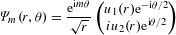where m is a half-integer. By inserting the ansatz into the eigenvalue equation we obtain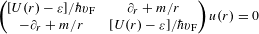To make direct connection with the STS measurements we calculate the local density of states LDOS(ɛ) as a function of r. The LDOS can be written as the sum of m-state contributions, LDOS(ɛ) = ∑mLDOSm(ɛ), with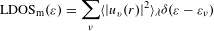where ν labels the radial eigenstates for fixed m and 〈|uv(r)|2λ = ∫0dr′|uν(r′)|2${\text{e}}^{–{\left(r–{r}^{\prime }\right)}^{2}/2\lambda }$ represents a spatial average of the wavefunction centred at r with a Gaussian weight λ/r = 0.01. To solve the radial Dirac equation, we use the finite difference method discretized in 1,200 lattice sites in the interval 0 < r < L, with a large repulsive potential at r = L = 12r. Spurious states arising from the finite potential jumps at the boundaries, localized within a few lattice sites of r = 0 and r = L, are excluded. We sum over eigenstates with azimuthal quantum numbers −401/2 ≤ m ≤ 401/2, which is sufficient to accurately represent the states in the energy range of interest. The delta function is approximated as a Lorentzian with width 0.3ɛ, which is sufficiently small that the intrinsic linewidths of the quantum dot eigenstates are preserved.

### Data availability.

The data that support the plots within this paper and other findings of this study are available from the corresponding author on reasonable request.